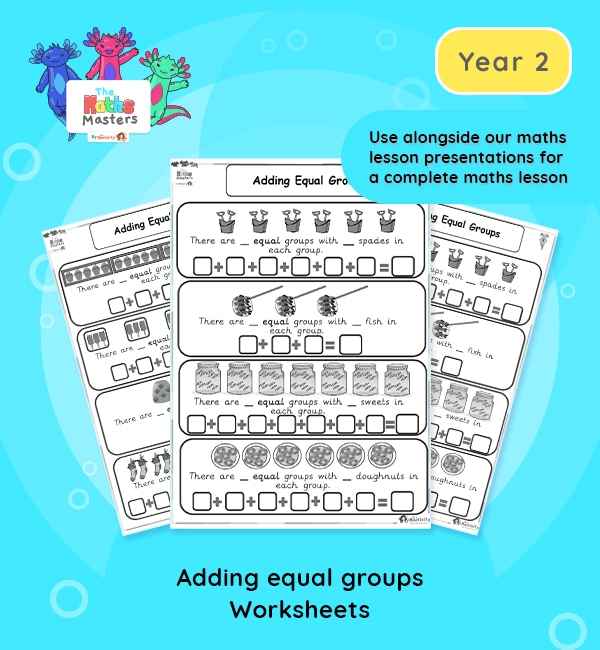# Year 2 | Adding Equal Groups Worksheets## Year 2 multiplication worksheets

Aligned with the maths mastery approach, these Year 2 | Adding Equal Groups Worksheets are designed to be used alongside our Year 2 | Adding Equal Groups Lesson Presentation for a complete maths lesson. The maths worksheets requires children to add equal groups in preparation for understanding multiplication. The worksheets can also be cut up and used individually in children’s books to demonstrate knowledge and progression, without the need for a whole worksheet.

Topic: Multiplication and division

This lesson practises adding equal groups of 2s, 5s, 10s and 3s using repeated addition. Children also have learnt how to add three 1-digit numbers, so they can also add up to three equal groups of up to 9 objects.

It builds on learning of adding equal groups from the end of the autumn term.

NC Links: Recall and use multiplication and division facts for the 2,5, and 10 times tables. *solve problems involving multiplication and division using materials, array, repeated addition, mental methods and multiplication and division facts including problems in context. *Count in steps of 2, 3, 5, and 10.

TAF Statements: Working At  – Recall and use multiplication and division facts for 2, 5, and 10 and use them to solve simple problems.

Greater Depth – Use reasoning about number and relationships to solve more complex problems and explain their thinking.

Year 1 conceptual prerequisites: Count in multiples of 2, 5 and 10

## Recently Viewed